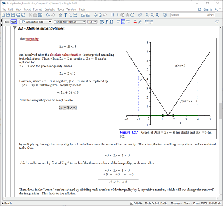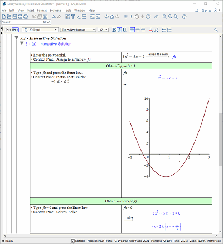Circle Triangle - Maple Help

Circle Triangle

 Main Concept Suppose you choose three distinct points on the circumference of a circle. No matter which three points you choose, you will always be able to form a triangle, but the size and shape will vary depending on how close or how far the points are away from each other; some triangles will be acute, some will be obtuse, and some will even be right-angled.   If the points are chosen at random, the theoretical probability that they form an acute triangle is approximately $\frac{1}{4}$ or 25%.  To see how well this theoretical probability works in practice, select three points along the circumference of the circle below at random by clicking on the image. As you select more points, more sides of the triangle will be drawn automatically, and the type of the triangle formed will be displayed, along with the overall data history, once the third point is selected. To create another triangle manually, click the Start a new triangle button. You can continually select your own random points each time, or let Maple randomly select points for you by clicking the Pick a random triangle button. To have Maple randomly generate $n$ triangles, enter a value for and then click the Pick n random triangles button.n =



 More MathApps